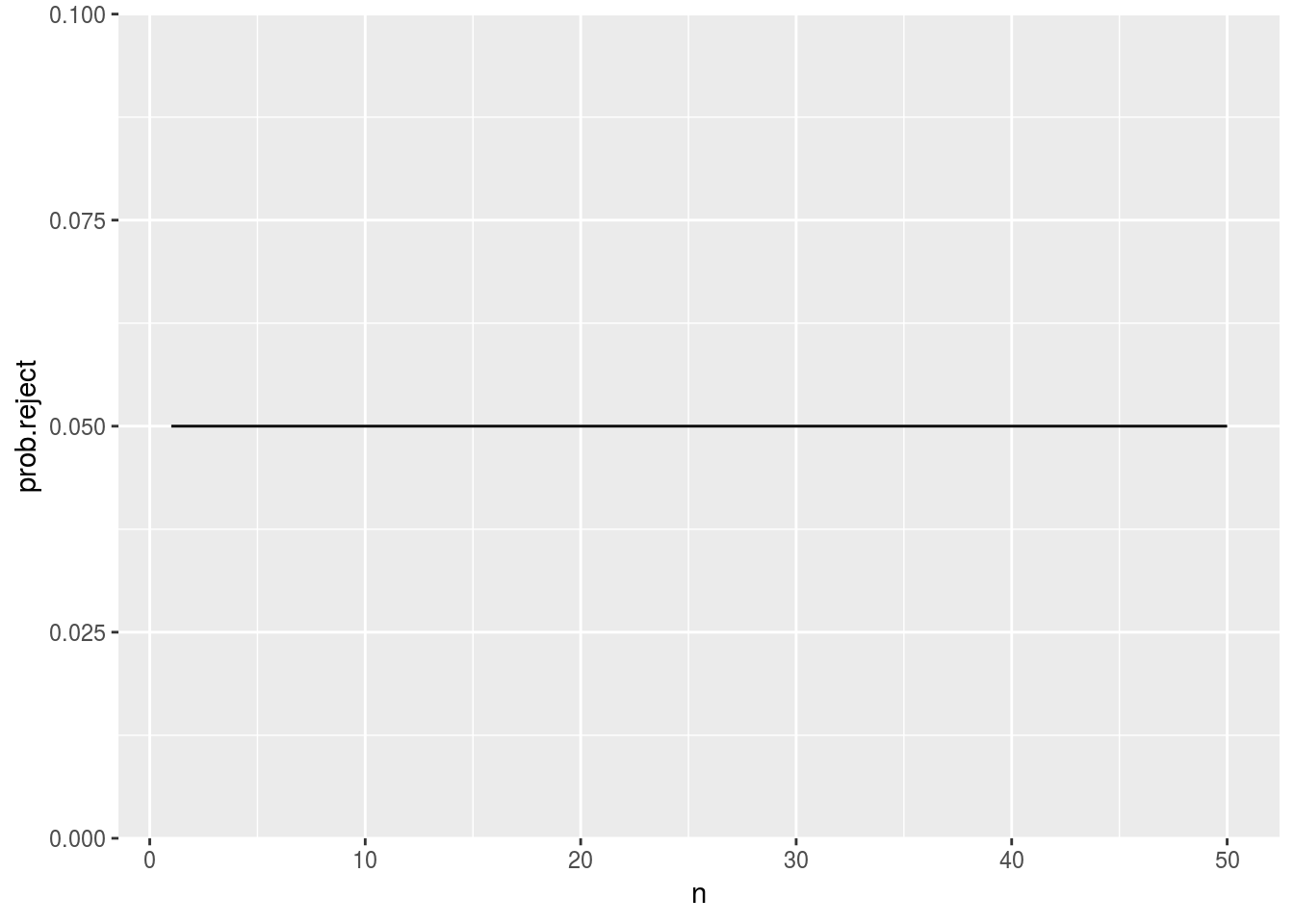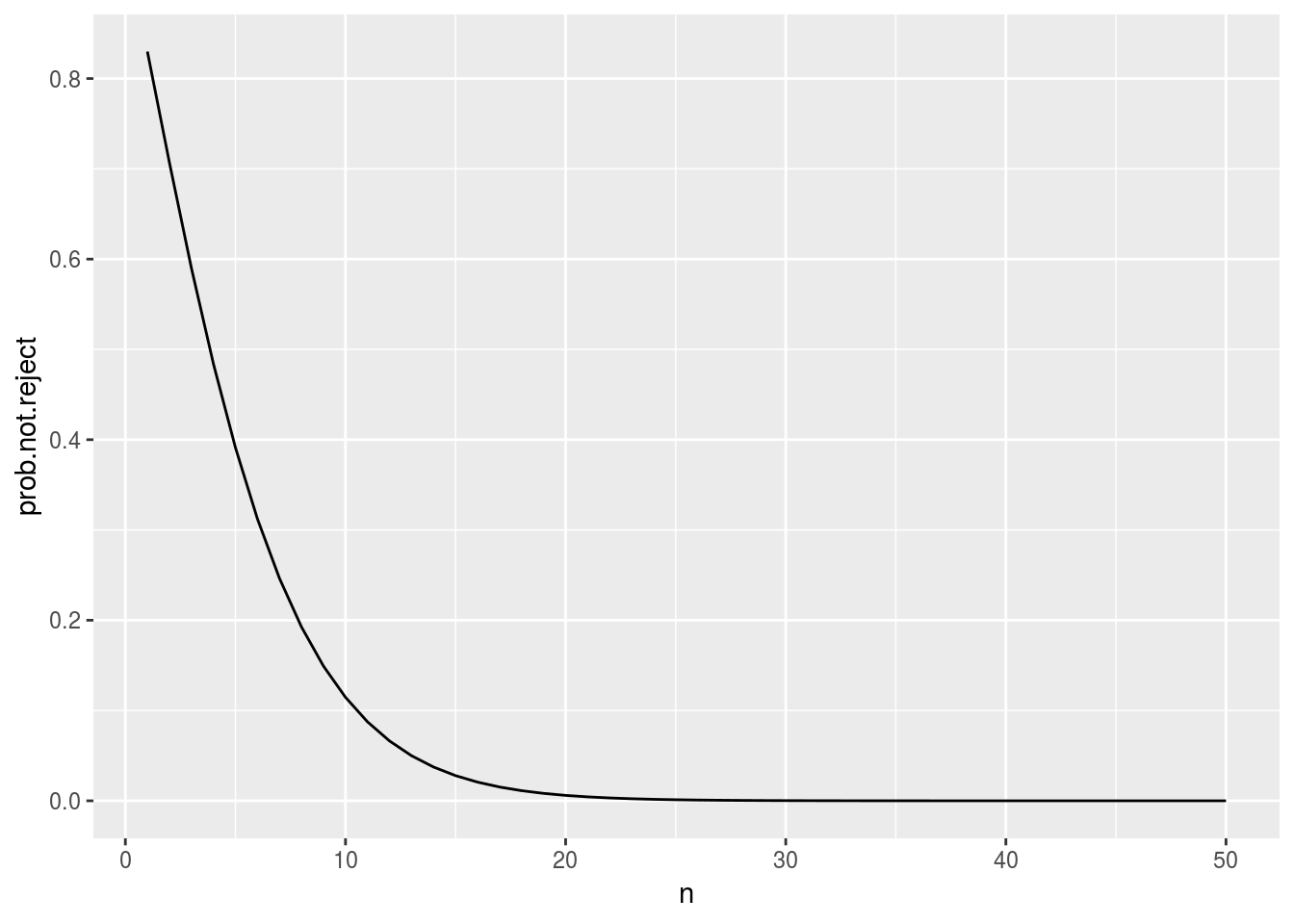### Question 1(a)

A dataframe gene.df contains the gene expression levels for 7000 genes, for 50 tumors. Each column in the dataframe (except the last one) corresponds to a genes, and each row corresponds to a tumor. Entries in the last column in the dataframe read "AML" if the tumor is an AML tumor, and ALL if the tumor is an ALL tumor.

   X1 X2 ... X7000 Class
1 100 20 ...    50   AML
2  20 10 ...    20   ALL
3  40  0 ...     0   ALL
4  20 30 ...    30   AML
...
...
50 10 15 ...    35   AML

We can use the t-statistic to measure the difference between the distributions of gene expression levels for ALL and AML tumors for each gene. Write code that finds the largest such t-statistic in the dataset described above.

#### Solution

t.stat.col <- function(col.i, gene.df){
ALL <- gene.df[gene.df$Class == "ALL", col.i] AML <- gene.df[gene.df$Class == "AML", col.i]
return(t.test(ALL, AML)$statistic) } all.t.stats <- sapply(1:(ncol(t.stat.col)-1), FUN = t.stat.col, gene.df) largest.t <- max(all.t.stats) Note: using the formula for the t-statistic is also fine. ### Question 1(b) Suppose we have pre-registered a null-hypothesis about gene #50 in the data frame (i.e., column X50): the mean gene expression levels do not differ between ALL and AML tumors. Explain the steps needed to test this hypothesis. Include code that you would use to compute any quantities needed. Explain what visualizations would be needed. State how to proceed after the visualization, and what conclusions can be drawn from the visualization and from the numbers computed. Use the 5% threshold. If you need the degrees of freedom parameter, you can assume it is nu. Be as specific as possible. #### Solution 1. Make sure that the model assumptions hold: display the histograms and/or boxplots for the expression levels in both the ALL and AML sets for gene #50. The histograms should be bell-shaped and symmetric. The boxplots should be symmetric, and there should be few, if any, outliers. We cannot proceed unless the model assumptions are satisfied. 2. If we are satisfied that the model assumptions are not violated, we can compute the t-statistic: ALL <- gene.df[gene.df$Class == "ALL", 50]
AML <- gene.df[gene.df\$Class == "AML", 50]

t.stat <- (mean(ALL)-mean(AML))/(sqrt(sd(ALL)^2/length(ALL) + sd(AML)^2/length(AML)))

Finally, we can compute the p-value:

2 * pt(-abs(t.stat), df = nu)

If the p-value is less than a threshold, say 5%, we can reject the null-hypothesis that the ALL and AML tumors do not differ in terms of the gene expression levels for gene # 50.

(Note: using t.test is fine too)

### Question 1(c)

What is the problem with testing $$7000$$ hypotheses, one for each gene, that the ALL and AML gene expression levels are the same?

#### Solution

The problem is that testing more than one hypothesis inflates the probability of false discovery. If we test just one pre-registered hypothesis, we have just 5% of rejecting a true null hypothesis (if we use 5% as the threshold for rejecting the null hypothesis). If we test 7000 hypotheses, we are almost certain to reject some true null hypotheses.

### Question 2

A random sample of 150 students was asked whether they prefer tea or coffee. 60 students said they preferred tea, and 90 students said they preferred coffee. Write code to test the hypothesis that 67% of students prefer coffee (with a 5% threshold). Use pbinom. Clearly indicate how to use the output of the code you wrote to make a conclusion.

#### Solution

First, we need to compute the p-value.

One possibility is to compute:

2 * pbinom(q = 90, size = 150, prob = .67)
##  0.08554224

Another acceptable possiblity is:

pbinom(q = 90, size = 150, prob = .67) + (1 - pbinom(q = round(.67*150 + (.67*150-90)) - 1, size = 150, prob = .67))
##  0.08200596

If the p-value is small than 0.05, we would reject the null hypothesis that 67% prefer coffee. Otherwise, we wouldn’t.

### Question 3

You are auditing a classifier for fairness with respect to demographic A and demographic B. You are specifically interested in false positive parity. Your test set consists of 100 people from demographic A, and 100 people from demographic B. The labels (1’s and 0’s) for the test sets are stored in the vectors testA and testB, respectively. The predictions of the classifier are stored in predA and predB, respectively. (The people are assumed to be in the same order in both sets of vectors.) Write code that tests the hypothesis that false positive parity is satisfied, and outputs the conclusion. Include comments that explain what you are doing (this is especially important for situations where the solution is a little wrong and we need to figure out how to assign partial credit). You must use fake-data simulation to generate fake data from demographics A and B in order to solve the problem. Use the 5% threshold.

#### Solution

Let’s define testA, testB, predA, and predB (this was not necessary on the test)

testA <- c(rep(1, 50), rep(0, 50))
predA <- c(rep(1, 40), rep(0, 50), rep(1, 10))

testB <- c(rep(1, 50), rep(0, 50))
predB <- c(rep(1, 40), rep(0, 55), rep(1, 5))

Now, let’s write a function that computes the FPR

FPR <- function(test, pred){
return(sum(test == 0 & pred == 1)/sum(test == 0))
}

Now, let’s compute the FPRs for set A and set B:

FPR.A <- FPR(testA, predA)
FPR.B <- FPR(testB, predB)

Now, let’s write a function that generates fake label data and returns the difference between the FPRs in the fake data. We’ll just generate fake label data for the negative samples – we could just copy the labels for the positive samples from the original dataset, if we wanted to, but that would make things less clear.

fake.neg.pred <- function(n.neg, fpr){
# Generate the predictions for n.neg examples that are negative,
# where the false positive rate is fpr
# Return the false positive rate in the fake data
neg.pred <- rbinom(n = n.neg, size = 1, prob = fpr)
return(mean(neg.pred))
}

fake.neg.pred.diff <- function(testA, testB){
# Figure out the number of negative examples in testA and testB, and
# then generate fake data where the underlying FPR is the same
n.neg.A <- sum(testA == 0)
n.neg.B <- sum(testB == 0)
fpr <- (FPR.A + FPR.B)/2
return(fake.neg.pred(n.neg.A, fpr) - fake.neg.pred(n.neg.B, fpr))
}

We are now ready to compute the p-value:

mean(abs(replicate(10000, fake.neg.pred.diff(testA, testB))) > abs(FPR.A-FPR.B))
##  0.1217

### Question 4(a)

Sketch a graph where the x axis is the sample size of a sample from a normal distribution with a mean 0, and the y-axis is the probability of making a type I error when testing the hypothesis that the mean is 0. Explain what a type I error is, and why the shape of the graph is what it is.

#### Solution

A type I error occurs when a true null hypothesis is rejected. If the null hypothesis is true, the probability of a type I error is always 5%, so the graph is flat.

n <- 1:50
prob.reject = rep(0.05, 50)
df <- data.frame(n = n, prob.reject = prob.reject)
ggplot(df, mapping = aes(x = n, y = prob.reject)) +
geom_line()(ggplot code was not required. We accepted answers where the answer stabilized at 5% very fast with minimal penalty.)

### Question 4(b)

Sketch a graph where the x axis is the sample size of a sample from a normal distribution with a mean different from 0, and the y-axis is the probability of making a type II error when testing the hypothesis that the mean is 0. Explain what a type II error is, and why the shape of the graph is what it is

#### Solution

A type II error occurs when a false null hypothesis is not rejected. For small datasets, there would not always be enough evidence to reject even a false null hypothesis. With large enough amounts of data, even an approximately true hypothesis would always be rejected.

# OPTIONAL: only the shape of the curve was needed
n <- 1:50

# The lower end of the region where the p-value is > 5%
# If the sample mean is in [-q, q], we do not reject the null hypothesis
q <- qnorm(p = 0.025, mean = 0, sd = 1/sqrt(n))

# MORE ADVANCED: if we think we are performing a t-test, we would use
# n <- 2:50
# q <- qt(p = 0.025, df = n - 1)/sqrt(n)

prob.not.reject <- pnorm(abs(q), mean = 1, sd = 1/sqrt(n)) - pnorm(-abs(q), mean = 1, sd = 1/sqrt(n))
###################################################

df <- data.frame(n = n, prob.not.reject = prob.not.reject)
ggplot(df, mapping = aes(x = n, y = prob.not.reject)) +
geom_line()(ggplot code was not required. Note that the curve would be somewhat different for different true hypotheses.)

### Question 5

Explain the claim that all null hypotheses in the social and life sciences are false, and that therefore type I and type II errors are impossible.

#### Solution

The claim relies on the idea that, outside of (perhaps) physics, no null hypothesis is ever exactly true: there will be always be (at least extremely tiny) differences between any two groups of tumors, populations, countries, etc.

If we accept this claim, type I errors (rejecting a true null hypothesis) is impossible since no null hypothesis is true. Type II errors are impossible since if we recognize that no null hypothesis is true, so that we would never accept a null hypothesis as true; and failing to reject a null hypothesis is not an error at all anyway.

See the Andrew Gelman blog post we discussed in lecture https://statmodeling.stat.columbia.edu/2004/12/29/type_1_type_2_t/